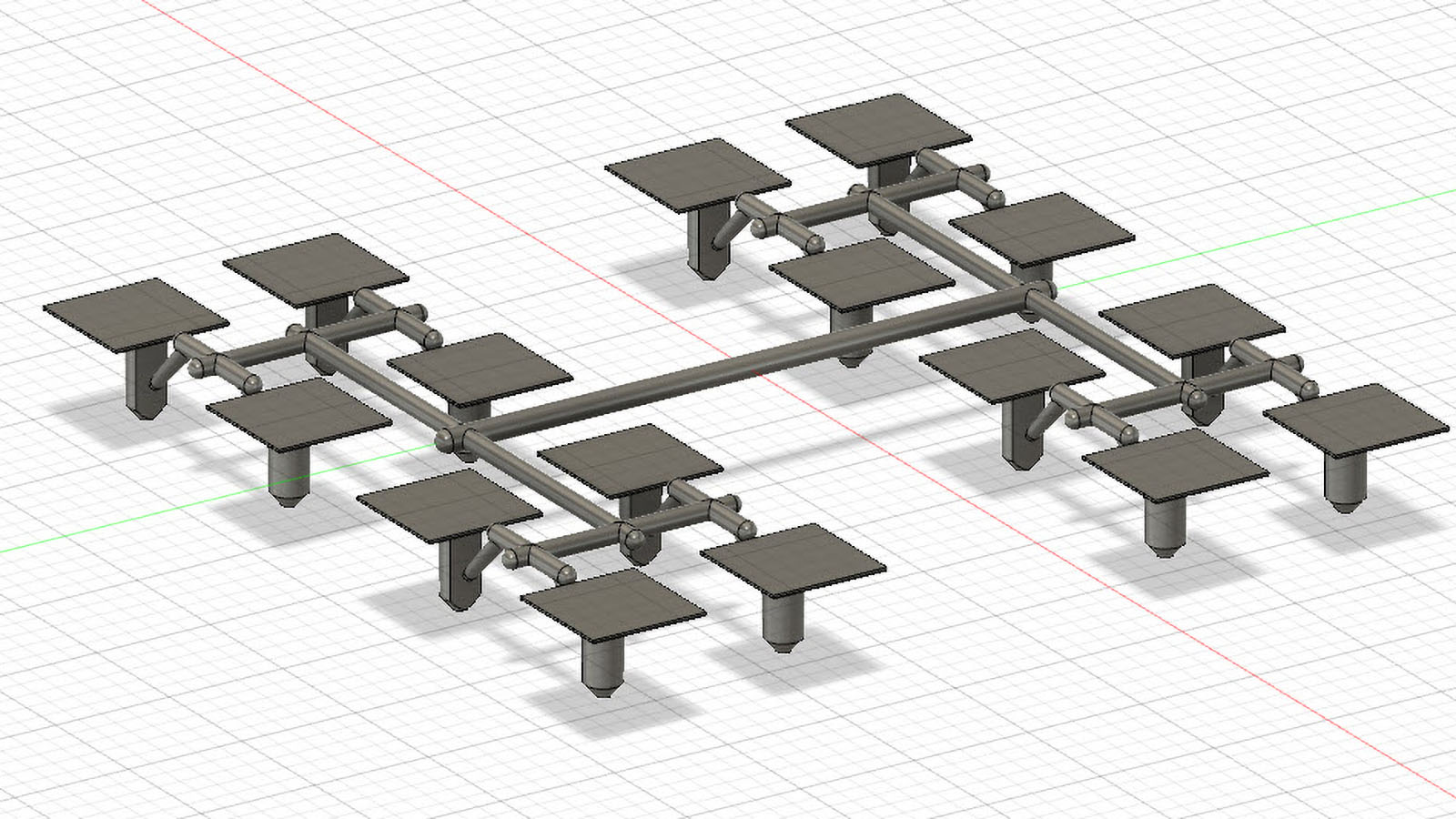# Understanding the Cavitation Cost Benefit Analysis

Cavitation refers to the number of parts a mold makes on each cycle. When starting a new project, there’s going to be a give and take between the additional tooling expense related to adding cavitation vs. the long term savings in the piece price. Sometimes it’s an obvious choice and other times, not so much.

The primary benefit of multi-cavity tooling is the optimization of machine time. Molding machines cost a certain amount per hour to run whether they produce one part per hour or thousands.

For example, a mold producing a single plastic part may have a 20 second cycle time. Making a two-cavity mold may yield little or no additional machine time, keeping the cycle at 20 seconds. A four cavity could be 22 second cycle time, 8 cavity maybe a 24 second, and a 16 maybe a 26 second. Here’s the math below showing the savings if we used a \$40 per hour machine rate, changing the cavitation and related cycle time. Note this is not factoring all other variables like plastic cost and packaging.

(Machine Rate) / (((3600 Seconds in 1 hour) / (Cycle Time)) X (cavitation))

Single Cavity Machine Cost Per Part = \$0.222

Two Cavity Machine Cost Per Part = \$0.111

Four Cavity Machine Cost Per Part = \$0.061

Eight Cavity Machine Cost Per Part = \$0.033

Sixteen Cavity Machine Cost Per Part = \$0.018

In that basic example, the advantage is obvious. So let’s take a look at a simple return on investment factoring in the additional tooling cost that could be related to adding cavitation. Let’s say the tooling costs are the following.

Single Cavity Mold Cost= \$5,000

Two Cavity Mold Cost= \$7,500

Four Cavity Mold Cost= \$12,500

Eight Cavity Mold Cost= \$20,000

Sixteen Cavity Mold Cost= \$40,000

Let’s say your project would require 120,000 parts per year. The numbers below are comparing the ROI at each increase in cavitation compared to the previous level of cavitation. In other words, a two-cavity is compared to the single cavity, the four to the two, and so on. The ROI time is followed by total savings over a five years after the return on investment is complete.

Two Cavity = 2.2 Month ROI / Total Savings in five years = \$64,170

Four Cavity = 10 Month ROI / Total Savings in five years = \$31,670

Eight Cavity = 25 Month ROI / Total Savings in five years = \$12,500

Sixteen Cavity = ROI Over 5 years

Let’s look at those same numbers if the annual volume was 2,500,000

Two Cavity = 0.1 Month ROI / Total Savings in five years = \$1,386,390

Four Cavity = 0.5 Month ROI / Total Savings in five years = \$758,890

Eight Cavity = 1.3 Month ROI / Total Savings in five years = \$409,160

Sixteen Cavity = 6.3 Month ROI / Total Savings in five years = \$205,695

You can see how critical it can be to estimate annual volume accurately and balance the cost-benefit of increasing cavitation. Looking at total machine time in higher volume applications, lower cavitation may not even be feasible. At 2.5 million parts per year, the single cavity mold would take over 500 days to run, and the two-cavity would take 289 days out of the year. Unfortunately, the calculations are not always that easy.

Part designs requiring cam actions, hydraulics, and other features can get significantly larger when adding cavitation. Because of this, a larger press size may be required, which will cost more per hour to run. Larger molds may also require a hot runner system, which can significantly increase the cost of the mold. You can see that the cost-benefit analysis should be done on a case by case basis.

Deciding on cavitation when starting a molding project can be a delicate balance of upfront tooling cost and piece price. What may seem obvious on the surface may not be once you understand what goes into the molding process. This is an important step in planning your molding project, and your molder should present you with the options to best suit your application.OUR LOCATION